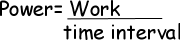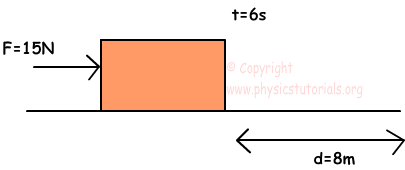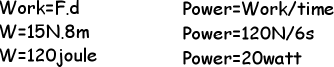Power

POWER

Power is the rate of work done in a unit of time. It can be misunderstood by most of the students. They think that more power full machine does more work. However, power just shows us the time that the work requires. For example, same work is done by two different people with different time. Say one of them does the work in 5 seconds and the other does in 8 seconds. Thus, the man doing same work in 5 seconds is more power full. The shorter the time the more power full the man. Let’s represent it mathematically;The unit of the power from the equation given above, joule/s, however, we generally use the unit of power as watt.

1joule/s=1watt

Example: Find the power of the man who pushes the box 8m with a force of 15N in a 6seconds.The power of the man is 20 watt. In other words he does 20 joule work in 6 seconds.Amount of power does not show the amount of work done. It just gives the time that work requires.

Work Power Energy Exams and Solutions

Author: